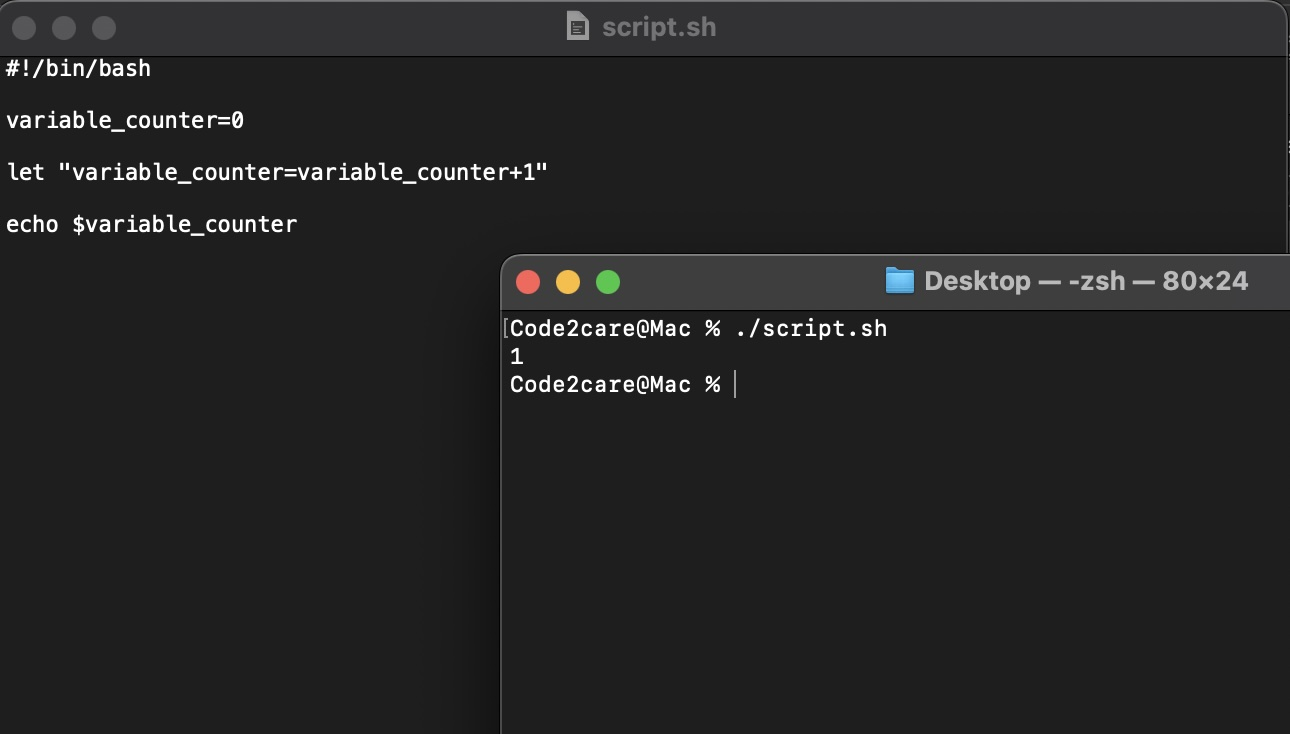# Ways to Increment a counter variable in Bash Script

If you are writing a bash script and you want to increment a counter variable, below are five ways in which you can do it.

## Option 1: Using += operator

``````#!/bin/bash

counter_variable=0

((counter_variable+=1))

echo \$counter_variable ``````

Output:

1

## Option 2: Using let command

``````#!/bin/bash

counter_variable=0

let "counter_variable=counter_variable+1"

echo \$counter_variable ``````

Output:

1

## Option 3: Using arithmetic expansion

``````#!/bin/bash

counter_variable=2

((counter_variable++))

echo \$counter_variable ``````

Output:

3

## Option 4: Using expr command

``````#!/bin/bash

counter_variable=5

counter=\$(expr \$counter_variable + 1)

echo \$counter_variable ``````

Output:

6

## Option 5: Using (( )) construct

``````#!/bin/bash

counter_variable=9

((counter_variable++))

echo \$counter_variable ``````

Output:

10Documentation/Man Pages:

1. `let` command: Bash `let` command

2. Arithmetic expansion: Bash Arithmetic Expansion

3. `expr` command: Bash `expr` command

4. `+=` operator: Bash Shell Arithmetic

5. `(( ))` construct: Bash Shell Arithmetic

-

### Facing issues? Have Questions? Post them here! I am happy to answer!Author Info:

Rakesh is a seasoned developer with over 10 years of experience in web and app development, and a deep knowledge of operating systems. Author of insightful How-To articles for Code2care.